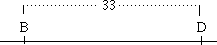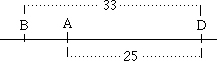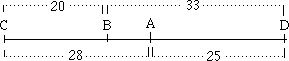# Campbell Problem 11

Molecular Genetics Problem 11
11. Determine the sequence of genes along a chromosome based on the following recombination frequencies: A-B, 8 map units; A-C, 28 map units; A-D, 25 map units; B-C , 20 map units; B-D, 33 map units.

To solve this type of genetics problem choose the greatest map distance (in this case 33) and place the genes involved at opposite ends of a line representing a portion of the chromosome in question.

Note: the largest possible map distance is 50 representing 2 genes found at exact opposite ends of a chromosome. Genes found at opposite ends of a chromosome behave as if they are on different chromosomes.

Why? ______________________________________________

B-D = 33 map units so:Now choose a gene combination with either B or D in it. For example:

A-D = 25 map units:Two combinations remain: A-C = 28 and B-C = 20. Where to put the C-gene? Since:

a) the distance from A to B is about 8 (see above) and b) the distance from C to B is less than the distance from A to B

the C-gene must be to the left of the B-gene. So:The problem  with this diagram is: the map distance between any two genes cannot exceed 50! According to our solution the C-D distance is 28+25 or 53!

BACK